## Cardioid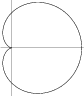The curve given by the Polar equation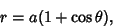(1)

sometimes also written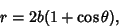(2)

where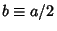, the Cartesian equation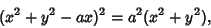(3)

and the parametric equations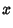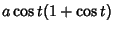(4)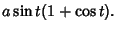(5)

The cardioid is a degenerate case of the Limaçon. It is also a 1-Cusped Epicycloid (with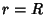) and is the Caustic formed by rays originating at a point on the circumference of a Circle and reflected by the Circle.

The name cardioid was first used by de Castillon in Philosophical Transactions of the Royal Society in 1741. Its Arc Length was found by La Hire in 1708. There are exactly three Parallel Tangents to the cardioid with any given gradient. Also, the Tangents at the ends of any Chord through the Cusp point are at Right Angles. The length of any Chord through the Cusp point is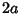.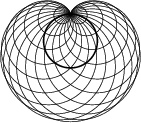The cardioid may also be generated as follows. Draw a Circle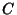and fix a pointon it. Now draw a set of Circles centered on the Circumference ofand passing through. The Envelope of these Circles is then a cardioid (Pedoe 1995). Let the Circlebe centered at the origin and have Radius 1, and let the fixed point be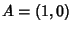. Then the Radius of a Circle centered at an Angle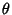from (1, 0) is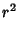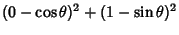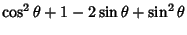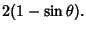(6)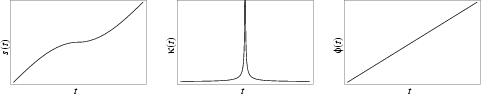The Arc Length, Curvature, and Tangential Angle are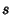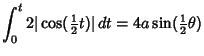(7)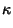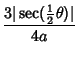(8)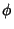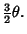(9)

As usual, care must be taken in the evaluation of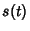for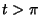. Since (7) comes from an integral involving the Absolute Value of a function, it must be monotonic increasing. Each Quadrant can be treated correctly by defining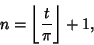(10)

where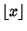is the Floor Function, giving the formula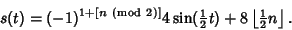(11)

The Perimeter of the curve is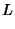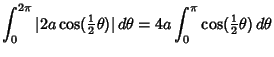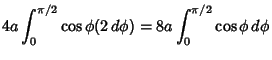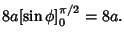(12)

The Area is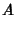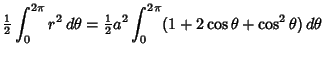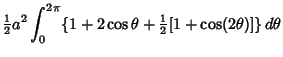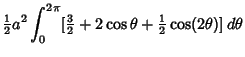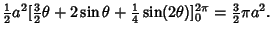(13)

References

Gray, A. Cardioids.'' §3.3 in Modern Differential Geometry of Curves and Surfaces. Boca Raton, FL: CRC Press, pp. 41-42, 1993.

Lawrence, J. D. A Catalog of Special Plane Curves. New York: Dover, pp. 118-121, 1972.

Lee, X. Cardioid.'' http://www.best.com/~xah/SpecialPlaneCurves_dir/Cardioid_dir/cardioid.html.

Lee, X. Cardioid.'' http://www.best.com/~xah/SpecialPlaneCurves_dir/Cardioid_dir/cardioidGG.html.

Lockwood, E. H. The Cardioid.'' Ch. 4 in A Book of Curves. Cambridge, England: Cambridge University Press, pp. 34-43, 1967.

MacTutor History of Mathematics Archive. Cardioid.'' http://www-groups.dcs.st-and.ac.uk/~history/Curves/Cardioid.html.

Pedoe, D. Circles: A Mathematical View, rev. ed. Washington, DC: Math. Assoc. Amer., pp. xxvi-xxvii, 1995.

Yates, R. C. The Cardioid.'' Math. Teacher 52, 10-14, 1959.

Yates, R. C. Cardioid.'' A Handbook on Curves and Their Properties. Ann Arbor, MI: J. W. Edwards, pp. 4-7, 1952.